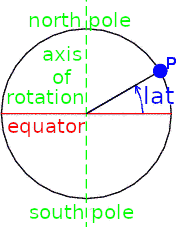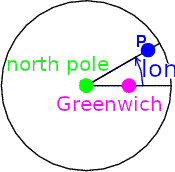﻿ Components of the "climate system"

How much air and water are on the earth, and where?

(This page is not finished. It will be revised after the lecture.)

Physical variables and SI units

Dimension of space, dimension of physical quantities ... different concept.

We live in 3-dimensional space, or 4-dimensional space-time.

3-dimensional space: position of a point can be expressed in 3 real numbers.

• linear orthogonal coordinate (Cartesian [=DesCartes] coordinate): 3 lengths (x, y, z)
• spherical cordinates (r, theta, phi)
• latitude, longitude, height ... variant of spherical coordinates. Note different conventionsUnit of spatial dimensions

• length: metre [m]
• Original concept: 1/107 of arc of a meridian from equator to pole
• Present definition: distance travelled by light in a certain number of seconds
• area: m2
• volume: m3

Unit of time

• SI unit: second [s]
• day = 24 * 60 * 60 s ["*" means multiplication here]
• year = approx. 365.25 days

Concepts of "weight" and "mass"

Primitive concept "weight", "heavy"

differentiated scientific concepts "gravity", "mass", "density"

Basic roles of mass in physical sciences

• Law of motion (Newton; formulated later): force = mass * acceleration
• Law of gravity (Newton): force = constant * mass * mass / distance2
• "Conservation of mass" (typically Lavoisier)

SI unit of mass and force

• Unit of mass: kilogram [kg] (= 1000 g)
• Original concept: mass of water of l litre (a 10 cm * 10 cm * 10 cm cube) at 4 deg. C
• Official definition was changed recently. (I will check references if you want.)
• Unit of force: newton [N] = kg m / s2
• Unit of pressure: pascal [Pa] = N / m2
• Unit of work, of energy: joule [J] = N m
• Unit of power, of energy flow per unit time: watt [W] = J / s

Conservation of mass

"Conservation" in physics is a different concept from the term in "conservation of cultural heritage" or "nature conservation".

No spontaneous generation, no spontaneous decay.

Formulation of conservation of X in isolated systems and open systems• In a system where there is no exchange of X across the boundary, X does not change in time.
• dX/dt = 0
• In a system where there are flows of X across the boundary (in a open system), X may change, but just because of net inflow of X.
• dX/dt = Fnet in = Fin - Fout
• If an open system is in a steady state where X does not change in time, net inflow of X is zero.
• 0 = Fnet in = Fin - Fout ... steady state

This concept of conservation is useful for "budget analysis", by which we can evaluate quantities which are not directly measurable.

Also, this concept of conservation is used for "prognostic" (i.e. forcasting-type) computations.

• ( X(t+Δt) - X(t) )/Δt = Fnet in
• X(t+Δt) = X(t) + Δt * Fnet in

The atmosphere

atmosphere vs. air

• air ... a (mixed) substance
• atmosphere ... a physical body (air surrounding a planet)

Vertical temperature profilethermosphere (ionosphere) ... ionized, not well mixed

troposphere, stratosphere, mesosphere ... electrically neutral, well mixed

Constituents of air

• well mixed gases: N2, O2, Ar, ... CO2 ...
• H2O in the troposphere
• O3 (ozone) in the stratosphere and other photochemically reactive gases
• aerosols (inhomogeneous)

expression of concentration (fraction)

• fraction by mass [kg/kg (non-dimensional); g/kg etc.]
• fraction by number of molecules, fraction by mol, "fraction by volume" (ideal gas assumption). [ppm, ppb, ppt]

2-component approximation of the atmosphere: "dry air" and water vapor

Atmospheric pressure

Unit: Pa = N/m2, hPa = 100 Pa

Sea level pressure : around 1000 hPa

(Approximate) hydrostatic balance

• pressure = g * {mass (per unit area) above}
• g : acceleration of gravity, approx. constant 9.8 m/s2
• 1000 hPa approx. corresponds to 10 t/m2, where "t" (tonne) is 1000 kg.
• layer-by-layer hydrostatic balance
• dp/dz = -ρ g
• p: pressure, ρ(rho) : density = mass/volume
• equation of state (ideal gas)
• p = ρ Rair T
• T: absolute temperature
• Rair (gas constant for air) = Runiv / Mair
• Runiv ... universal gas constant = Boltzmann constant * Avogadro constant
• Mair ... mass of air per mol [kg/mol] (corresponding to molecular weight, but note the unit!)
• If T is approximated as a constant, both p and ρ are proportional to exp(-z/H)
• H = Rair T / g

Approximate vertical distribution of pressure (also density)What proportion (in terms of mass) of the atmosphere is below 11 km (approx. in the troposphere)?

Density at 1000 hPa ... approx. 1.2 kg/m3 (Note: not g/m3)

Range of "specific humidity" (mass fraction of water vapor) at 1000 hPa ... 0 to 50 g/kg

Vertically integrated mass of water vapor per unit mass (global average)... 25 kg/m2

The Ocean

Ocean vs. seawater ... similar to atmosphere vs. air

Ocean covers 70 % of earth's surface

Maximum depth 11 km, average depth 4 km

Ingredients

• Water
• "Salt": ions Cl-, Na+, SO4(2-), Mg2+, ...
• dissolved gases (esp. oxygen)
• dissolved organic matter
• particulate matter

2-component approximation "water and salt"

"Salinity": mass of salt / mass of seawater. Typically 35 g/kg

Density of seawater

Density of water 1.0 g/cm3, 1000 kg/m3

At first approximation, density is constant.

For discussion of dynamics, variation of density due to tempearture and salinity is relevant.

Temperature dependence: higher temperature, lower density (thermal expansion).

Note: freshwater (salinity near zero) has abnormal characteristics "higher temperature, higher density" below 4 deg. C. Seawater (salinity near 35 g/kg) does not have this characteristics.

Salinity dependence: higher salinity, higher density.

Inhomogeneity of salinity is mainly caused by input/output of water (rather than input/output of salt).

• rainfall or inflow from rivers -> lower salinity
• evaporation from sea surface -> higher salinity
• formation of sea ice -> higher salinity of ambient water
• melting of sea ice -> lower salinity of ambient water

Vertical divisions and time scales of the ocean

(To be discussed after the energy balance of the climate system)

• (near-surface) mixed layer (very roughly 100 m) ... 1 year
• "upper ocean" (very roughly 500 m) ... tens of years
• "deep ocean" ... thousand years

Snow and ice ... the cryosphere

• ice on the sea
• sea ice ... frozen seawater
• containing salt, but less than in the water phase
• mostly seasonal (depth ca. 1 m) some multi-year (depth may be 10 m)
• icebergs ... ice cut off from glaciers
• "fresh" (nearly no salt)
• minor in terms of coverage of the earth
• ice on land (mostly "fresh")
• snowpack
• mostly seasonal (depth ca. 1 m)
• glaciers
• made from multi-year snowpack; flow (creep) by own weight, typical speed 10 m/year (cf. rivers 1 m/s)
• continental ice sheets
• Antarctica (depth ca. 3 km)
• Greenland
• (e.g. 20000 years ago, also in N America and N Europe)
• mountain glaciers
• Himalayas, Patagonia, Alaska, Alps, New Zealand ...
• ice below ground (including below seafloor)
• frozen ground (containing frozen soil water or frozen groundwater)
• permafrost (ground frozen all around the year)
• seasonally frozen ground

Water on the earth, hydrological cycle

[Figure from Oki (1999)]

• Taikan Oki [沖 大幹], 1999: The global water cycle. Global Energy and Water Cycles (K. A. Browning & R. J. Gurney, R.J. eds., Cambridge University Press) Section 1.2.

2019-Apr-25 (before lecture)
MASUDA Kooiti